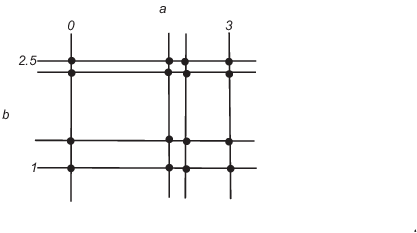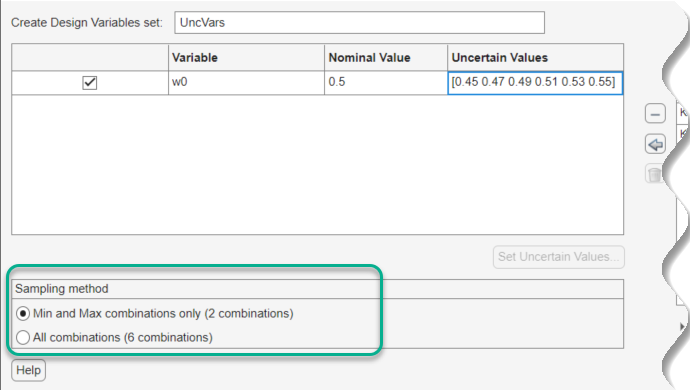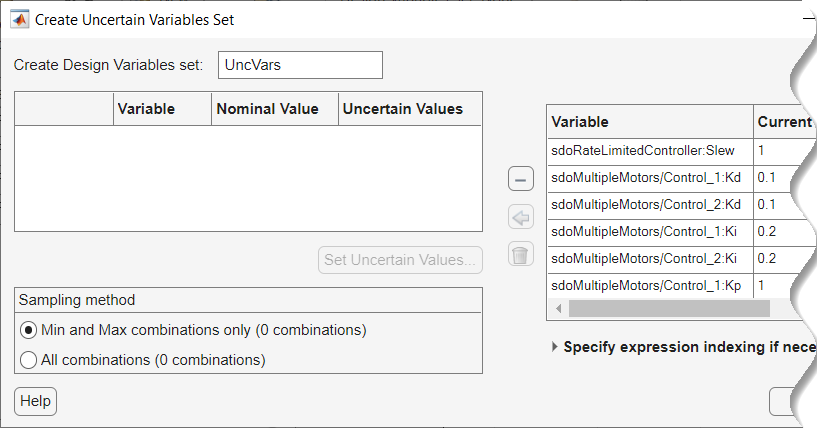## Optimizing Parameters for Robustness

### What Is Robustness?

A design is robust when its response does not violate design requirements under model parameter variations. Your model may contain parameters whose values are not precisely known. Such parameters vary over a given range of values and are defined as uncertain parameters. You may know the nominal value and the range of values in which these uncertain parameters vary.

You can use Simulink® Design Optimization™ software to incorporate the parameter uncertainty to test the robustness of your design. When you optimize parameters for robustness, the optimization solver uses the responses computed using all the uncertain parameter values to adjust the design variable values.

You can specify the same parameter both as a design and uncertain variable. However, you cannot use a parameter both as a design and uncertain variable in the same optimization run. Also, you cannot add uncertainty to controller or plant parameters during optimization-based control design in the Control System Designer.

The uncertain variables can be scalar, vector, matrix or an expression.

You can test and optimize parameters for model robustness in the following ways:

• Before Optimization. Specify the parameter uncertainty before you optimize the parameters to meet the design requirements. In this case, the optimization method optimizes the signals based on both nominal parameter values as well as the uncertain values. This mode requires more computational time.

• After Optimization. Specify the parameter uncertainty after you have optimized the model parameters to meet design requirements. You can then test the effect of the uncertain parameters by plotting the model's response. If the response violates the design requirements, you can optimize the parameters again by including the parameter uncertainty during the optimization.

### Sampling Methods for Uncertain Parameters

Sample values for uncertain parameters are a vector of numerical values. You can specify the vector yourself or generate a vector of random numbers using the software. The sample values you specify can be uniformly distributed or random. For example, four sample values for two uncertain parameters a and b in the range `[0 3]` and ```[1 2.5]``` may look like the following figure.There are two methods to determine the number of sample values to use during optimization:

• Only the combination of minimum and maximum values (circled)• Combination of the entire set of values (all solid dots in the previous figure)

Tip

Using only the minimum and maximum values during optimization increases the computation speed when compared to using the entire set of values.

For the previous example, there are 4 combinations using the minimum and maximum values and 16 combinations if you use all sample values.

In the Response Optimizer, you specify the sampling method using the options as shown in the following figure.### Optimize Parameters for Robustness (GUI)

This example shows how to optimize parameters for model robustness.

1. Load a saved Response Optimizer session.

```load sldo_model1_desreq_optim_sdosession; sdotool(SDOSessionData);```

The `sdotool` command opens the following Simulink model and a saved Response Optimizer session.The parameters of this model, `Kp`, `Ki` and `Kd`, have already been optimized to meet the following step response requirements:

• Maximum overshoot of 5%

• Maximum rise time of 10 seconds

• Maximum settling time of 30 seconds

2. Specify parameter uncertainty.

1. In the Uncertain Variables Set drop-down list, select New.A window opens where you specify uncertain variables.2. Click `w0` and `zeta` to select them.

3. Clickto add the selected parameters to an uncertain variables set.The software displays the following parameter settings:

• Variable — Parameter name

• Nominal Value — Nominal value of the parameters as specified in the Simulink model

• Uncertain Values — Values that the uncertain parameter can take. By default, the maximum and minimum values vary by 10% of the nominal value.

The total number of sample values to use during optimization is a combination of the maximum and minimum values of the uncertain parameters.

The check-box indicates that the parameter is included in the uncertain variable set. The default uncertain variable set name is `UncVars`.

Click . A new variable `UncVars` appears in Data area of the Response Optimizer.

3. Test the model robustness to the uncertain parameters.

1. Click Plot Model Response.The step response plot, displaying the requirements, updates.• The solid curve corresponds to the model response computed using the optimized parameters and nominal values of the uncertain parameter.

• The four dashed curves correspond to the model response with the minimum and maximum values of the uncertain parameters.

The dashed plot lines show that the response during the period of 10 to 20 seconds violates the design requirements.

4. Optimize the parameters for model robustness. Click .The Optimization Progress window opens which displays the optimization iterations.

After the optimization completes, the message ```Optimization converged``` indicates that the final model response computed by varying the uncertain parameters meets the specified design requirements.5. Examine the responses.

Tip

To view only the final responses of the model, right-click the white area in the plot and uncheck Responses > Show Iteration Responses.The final responses appear as the thick solid and dashed curves. The nominal and uncertain responses with parameter variations now meet the design requirements.

If your model contains referenced models, you can create an uncertain variable set using variables in the referenced models, using the Create Uncertain Variables Set dialog. For example, the first variable in the dialog box, `Slew`, is listed as `sdoRateLimitedController:Slew`. `sdoRateLimitedController` is the name of the referenced model with the variable `Slew`. The `Slew` variable has the same value for all instances of the `sdoRateLimitedController` model. In contrast, the variable `Kd` can have a different value for each instance of the referenced model containing it. For example, the second variable in the dialog box is listed as `sdoMultipleMotors/Control_1:Kd`. The upper-level model `sdoMultipleMotors` has block `Control_1`, which is a referenced model that has variable `Kd`. The value of this variable can be different than `Kd` in block `Control_2`, which is the third variable in the dialog box. To enable instance-specific values, `Kd` is specified as a model argument in the referenced model workspace.## Related Topics